Courses

# Test: Application Of Derivatives - 2

## 25 Questions MCQ Test Mathematics (Maths) Class 12 | Test: Application Of Derivatives - 2

Description
This mock test of Test: Application Of Derivatives - 2 for JEE helps you for every JEE entrance exam. This contains 25 Multiple Choice Questions for JEE Test: Application Of Derivatives - 2 (mcq) to study with solutions a complete question bank. The solved questions answers in this Test: Application Of Derivatives - 2 quiz give you a good mix of easy questions and tough questions. JEE students definitely take this Test: Application Of Derivatives - 2 exercise for a better result in the exam. You can find other Test: Application Of Derivatives - 2 extra questions, long questions & short questions for JEE on EduRev as well by searching above.
QUESTION: 1

### The function f (x) = x2 – 2 x is increasing in the interval

Solution: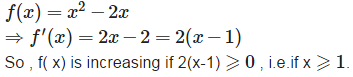QUESTION: 2

### The function f (x) = a x + b is strict increasing for all x ∈ R iff

Solution:

Since f ‘ (x) = a , therefore , f (x) is strict increasing on R iff a > 0.

QUESTION: 3

### Tangents to the curve x2+y2 = 2at the points (1, 1) and (– 1, 1)

Solution: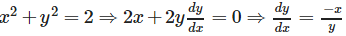therefore , slope of tangent at (1,1) = - 1 and the slope of tangent at (- 1 ,1) = 1. Hence , the two tangents in reference are at right angles.

QUESTION: 4

If x be real, the minimum value of x2−8x+17 is

Solution: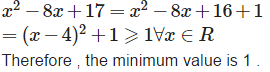QUESTION: 5

If a differentiable function f (x) has a relative minimum at x = 0, then the function y = f (x) + a x + b has a relative minimum at x = 0 for

Solution: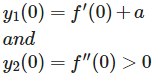And y has a relative minimum at x = 0 if f ‘(0)+a = 0 . a =0.

QUESTION: 6

The function f (x) = x2, for all real x, is

Solution: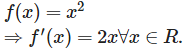Since f ‘(x) = 2x > 0 for x > 0,and f ‘ (x) = 2x < 0 for x < 0 ,therefore on R , f is neither increasing nor decreasing. Infact , f is strict increasing on [0 , ∞) and strict decreasing on (- ∞,0].

QUESTION: 7

The function f (x) = a x + b is strict increasing for all x ∈ R if

Solution:

Since f‘(x) = a , therefore , f (x) is strict increasing on R if f a > 0.

QUESTION: 8

Equation of the tangent to the curve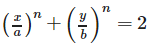at the point (a, b) is

Solution: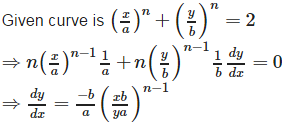Hence ,slope of tangent at (a , b) = -b/a . Therefore , the equation of tangent at (a , b ) is ;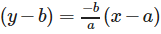QUESTION: 9

a log | x | + bx2 + x has its extreme values at x = – 1 and x = 2, then

Solution: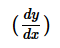= 0, at x = -1 and x = 2 i.e a + 2b = 1 and 4b + a/2 = -1. ⇒ a = 2 , b = -1/2

QUESTION: 10

Let f (x) be differentiable in (0, 4) and f (2) = f (3) and S = {c : 2 < c < 3, f’ (c) = 0} then

Solution:

Conditions of Rolle’s Theorem are satisfied by f(x) in [2,3].Hence there exist atleast one real c in (2, 3) s.t. f ‘(c) = 0 . Therefore , the set S contains atleast one element

QUESTION: 11

The function f (x) = m x + c where m, c are constants, is a strict decreasing function for all x ∈ R if

Solution:

f (x) = mx + c is strict decreasing on R
if f ‘ (x) < 0 i.e. if m < 0 .

QUESTION: 12

The function f (x) = x2−2x is strict decreasing in the interval

Solution:

f ‘ (x) = 2x – 2 = 2 (x - 1) < 0 if x < 1 i.e. x x∈ (−∞,1) x∈ (−∞,1). Hence f is strict decreasing in left decreasing in (−∞,1)

QUESTION: 13

The points on the curve 4 y = |x2−4| at which tangents are parallel to x – axis, are

Solution: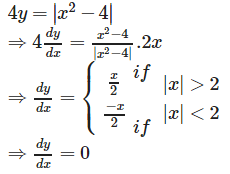Only when x = 0 and when x = 0 , y = 1. So, only at (0,1) tangent is parallel to x- axis.

QUESTION: 14

The maximum value of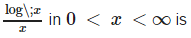Solution: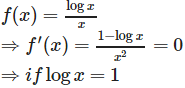i.e. x = e. Note that , f ‘(x) changes sign from positive to negative as we move from left to right through e. So, f (e) is maximum i.e. maximum value of f (x) is f (e)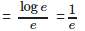QUESTION: 15

Every continuous function is

Solution:

Obviously, every differentiable function is continuous but every continuous function isn't differentiable.

QUESTION: 16

The function f(x) = tan−1x is

Solution: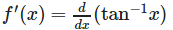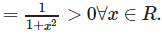Therefore , f is strictly increasing on R.

QUESTION: 17

For the curve x = t2−1,y = t2−t tangent is parallel to X – axis where

Solution: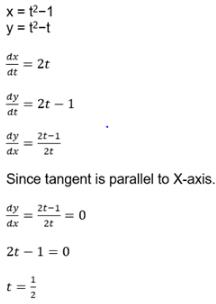QUESTION: 18

The slope of the normal to the curvex = a (cos θ + θ sin θ),y = a (sin θ – θ cos θ) at any point ‘θ’ is

Solution: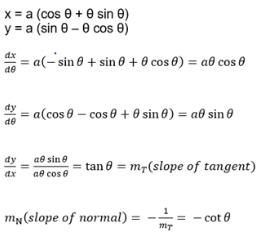QUESTION: 19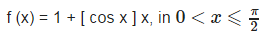Solution: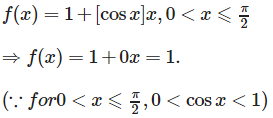QUESTION: 20

Rolle’s Theorem is not applicable to the function f(x) = | x | for −2⩽x⩽2 because

Solution: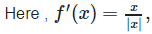which does not exist at x = 0 ∈ (-2 , 2). So , Rolle’s theorem is not applicable.

QUESTION: 21

Let f (x) = x3−6x2+9x+8, then f (x) is decreasing in

Solution: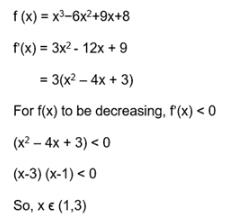QUESTION: 22

The equation of the normal to the curve y = sinx at (0, 0) is

Solution: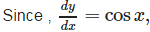therefore , slope of tangent at (0 , 0) = cos 0 = 1 and hence slope of normal at (0 , 0) is - 1 .

QUESTION: 23

The curve y = x1/5 has at (0, 0)

Solution: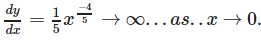so,at (0 ,0) , the curve y = x1/5 has a vertical tangent.

QUESTION: 24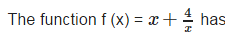Solution: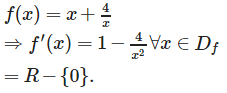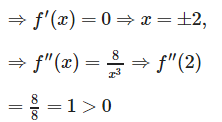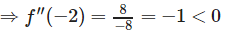So, f has a local minima at 2 and a local maxima at - 2 .

QUESTION: 25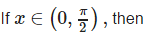Solution: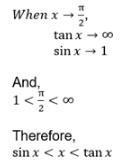Track your progress, build streaks, highlight & save important lessons and more!

### Similar Content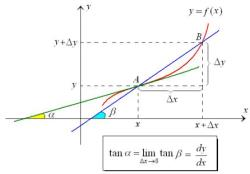### Related tests